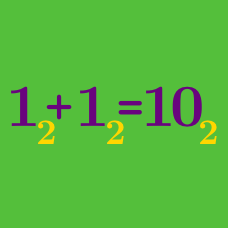Number Theory

# Integer Number Bases

Express the quaternary (4-ary) number $3 0 3 _4$ into a decimal number.

Convert the decimal number $98$ into a quinary (base-5) number.

Express a senary ($6$-ary) number $3 4$ into a decimal number.

Convert the decimal number $34$ into a ternary (base-3) number.

Express the decimal number $31$ into a septenary ($7$-ary) number.

×